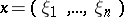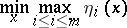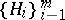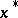# Chebyshev point

(diff) ← Older revision | Latest revision (diff) | Newer revision → (diff)

of a system of linear inequalitiesA pointat which the minimaxis attained. The problem of finding a Chebyshev point reduces to the general problem of linear programming .

A more general notion is that of a Chebyshev pointof a system of hyperplanesin a Banach space, i.e. a pointfor whichChebyshev points are often chosen as "solutions" of incompatible linear systems of equations and inequalities.

How to Cite This Entry:
Chebyshev point. Encyclopedia of Mathematics. URL: http://encyclopediaofmath.org/index.php?title=Chebyshev_point&oldid=14353
This article was adapted from an original article by Yu.N. Subbotin (originator), which appeared in Encyclopedia of Mathematics - ISBN 1402006098. See original article